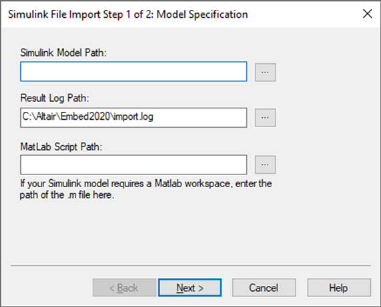## AFT

Category:  eDrives > Toolbox > Sensorless

Inputs:

Ialpha: Motor current in alpha-axis measured from ADC channels.

Ibeta: Motor current in beta-axis measured from ADC channels.

Valpha: Motor voltage in alpha-axis measured from ADC channels.

Vbeta: Motor voltage in beta-axis measured from ADC channels.

Outputs:

Cos: Cosine of the estimated angular position.

Sin: Sine of the estimated angular position.

Description: The AFT block tracks the flux vector while the drive is running. It estimates the rotor angular position and shaft speed. It also tracks and provides torque and flux of a motor while it is spinning in real time. Optionally, the AFT block can estimate rotor resistance for induction motors.#### Inverter Properties

Full Scale Current (A): Specifies the full-scale current value for normalization.

Full Scale Frequency (Hz): Specifies the full-scale frequency value for normalization.

Full Scale Voltage (V): Specifies the full-scale voltage value for normalization.

Voltage Filter (Hz): Specifies the corner frequency for low-pass voltage filters used for estimation and power calculation.

#### Motor Parameters

Flux (Wb): Specifies the estimated or calculated magnetic flux for one phase.

Rs Estimation Current (A): Specifies a DC injection current, which is introduced to the d-axis current for estimation of stator resistance.

Speed Filter (Hz): Specifies the corner frequency for the speed estimator inside the AFT.

Stator Inductance-Lq (H): Specifies the measured q-axis stator inductance of the motor.

Stator Resistance Rs (ohms): Specifies the measured stator resistance of one motor phase.

Rs Estimation scale: Specifies an empirical scale, which calibrates the estimator close to the stator resistance. Change this value to obtain an Rs max value close to the actual measured stator resistance.